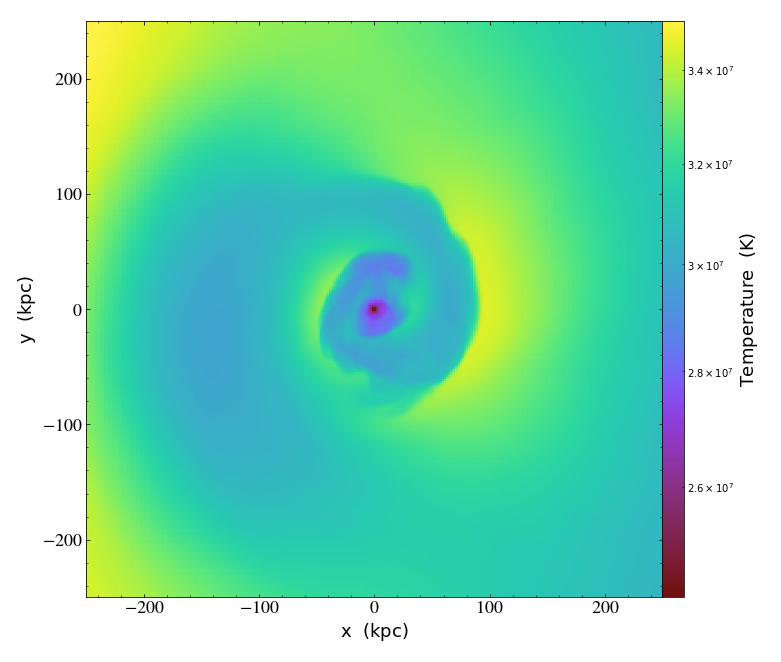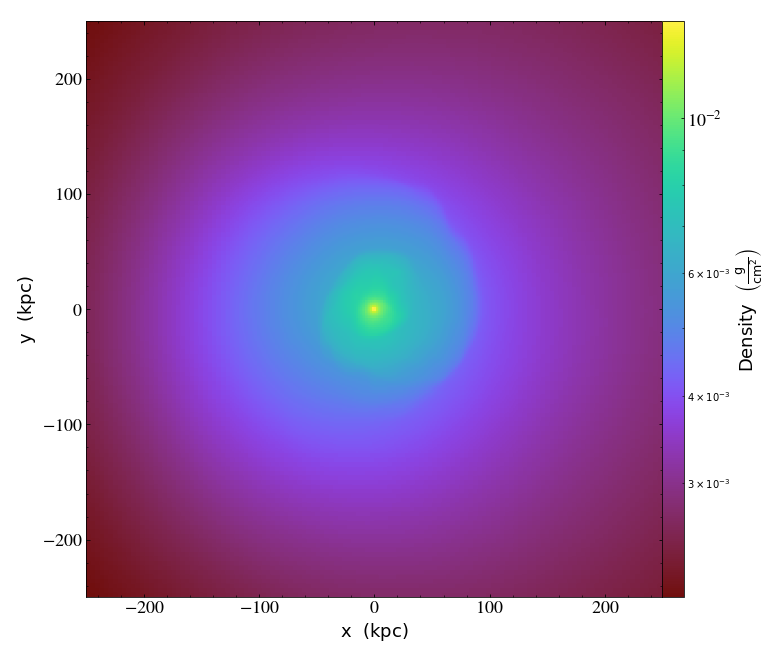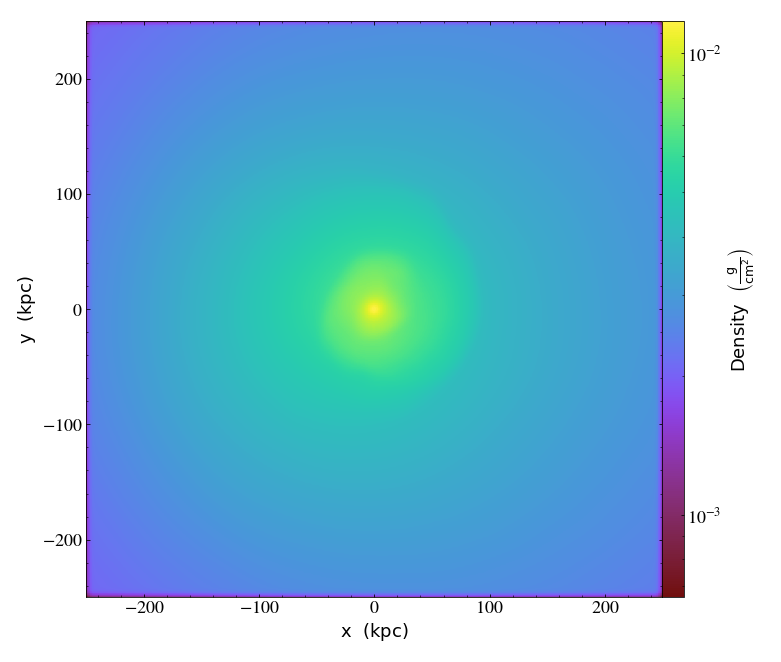# Writing FITS Images¶

Notebook

yt has capabilities for writing 2D and 3D uniformly gridded data generated from datasets to FITS files. This is via the FITSImageData class. We'll test these capabilities out on an Athena dataset.

In :
import yt

In :
units_override = {"length_unit": (1.0, "Mpc"),
"mass_unit": (1.0e14, "Msun"),
"time_unit": (1.0, "Myr")}


## Creating FITS images from Slices and Projections¶

There are several ways to make a FITSImageData instance. The most intuitive ways are to use the FITSSlice, FITSProjection, FITSOffAxisSlice, and FITSOffAxisProjection classes to write slices and projections directly to FITS. To demonstrate a useful example of creating a FITS file, let's first make a ProjectionPlot:

In :
prj = yt.ProjectionPlot(ds, "z", ("gas", "temperature"),
weight_field=("gas", "density"), width=(500., "kpc"))
prj.show()Suppose that we wanted to write this projection to a FITS file for analysis and visualization in other programs, such as ds9. We can do that using FITSProjection:

In :
prj_fits = yt.FITSProjection(ds, "z", ("gas", "temperature"), weight_field=("gas", "density"))


which took the same parameters as ProjectionPlot except the width, because FITSProjection and FITSSlice always make slices and projections of the width of the domain size, at the finest resolution available in the simulation, in a unit determined to be appropriate for the physical size of the dataset.

We can also set width manually in FITSProjection. For example, set the width to 500 kiloparsec to get FITS file of the same projection plot as discussed above.

In :
prj_fits = yt.FITSProjection(ds, "z", ("gas", "temperature"),
weight_field=("gas", "density"), width=(500., "kpc"))


## Making FITS images from Particle Projections¶

To create a FITS image from a particle field which is smeared onto the image, we can use FITSParticleProjection:

In :
dsp = yt.load("gizmo_64/output/snap_N64L16_135.hdf5")
prjp_fits = yt.FITSParticleProjection(dsp, "x", ("PartType1", "particle_mass"), deposition='cic')
prjp_fits.writeto("prjp.fits", overwrite=True)


Note that we used the "Cloud-In-Cell" interpolation method ("cic") instead of the default "Nearest-Grid-Point" ("ngp") method.

If you want the projection to be divided by the pixel area (to make a projection of mass density, for example), supply the density keyword argument:

In :
prjpd_fits = yt.FITSParticleProjection(dsp, "x", ("PartType1", "particle_mass"), density=True, deposition='cic')
prjpd_fits.writeto("prjpd.fits", overwrite=True)


## Using HDUList Methods¶

We can call a number of the AstroPy HDUList class's methods from a FITSImageData object. For example, info shows us the contents of the virtual FITS file:

In :
prj_fits.info()

Filename: (No file associated with this FITSImageData)
No.    Name      Ver    Type      Cards   Dimensions   Format     Units
0  TEMPERATURE    1 PrimaryHDU      29   (512, 512)   float64   K


We can also look at the header for a particular field:

In :
prj_fits["temperature"].header

Out:
SIMPLE  =                    T / conforms to FITS standard
BITPIX  =                  -64 / array data type
NAXIS   =                    2 / number of array dimensions
NAXIS1  =                  512
NAXIS2  =                  512
EXTEND  =                    T
EXTNAME = 'TEMPERATURE'        / extension name
BTYPE   = 'temperature'
BUNIT   = 'K       '
LUNIT   =                  1.0 / [kpc]
TUNIT   =                  1.0 / [Myr]
MUNIT   =    100000000000000.0 / [Msun]
VUNIT   =                  1.0 / [Mpc/Myr]
BFUNIT  =  0.02851538907227106 / [G]
TIME    =             2700.111
WCSAXES =                    2
CRPIX1  =                256.5
CRPIX2  =                256.5
CDELT1  =            0.9765625
CDELT2  =            0.9765625
CUNIT1  = 'kpc     '
CUNIT2  = 'kpc     '
CTYPE1  = 'LINEAR  '
CTYPE2  = 'LINEAR  '
CRVAL1  =                  0.0
CRVAL2  =                  0.0
LATPOLE =                 90.0
WCSNAME = 'yt      '
MJDREF  =                  0.0                                                  

where we can see that the units of the temperature field are Kelvin and the cell widths are in kiloparsecs. Note that the length, time, mass, velocity, and magnetic field units of the dataset have been copied into the header

If we want the raw image data with units, we can use the data attribute of this field:

In :
prj_fits["temperature"].data

Out:
unyt_array([[34403626.80544101, 34403626.80544101, 34403626.80544101,
..., 30453524.91959378, 30453524.91959378,
30453524.91959378],
[34403626.80544101, 34403626.80544101, 34403626.80544101,
..., 30453524.91959378, 30453524.91959378,
30453524.91959378],
[34403626.80544101, 34403626.80544101, 34403626.80544101,
..., 30453524.91959378, 30453524.91959378,
30453524.91959378],
...,
[35071597.64056484, 35071597.64056484, 35071597.64056484,
..., 30673962.05243628, 30673962.05243628,
30673962.05243628],
[35071597.64056484, 35071597.64056484, 35071597.64056484,
..., 30673962.05243628, 30673962.05243628,
30673962.05243628],
[35071597.64056484, 35071597.64056484, 35071597.64056484,
..., 30673962.05243628, 30673962.05243628,
30673962.05243628]], 'K')

### Changing Aspects of the Images¶

We can use the set_unit method to change the units of a particular field:

In :
prj_fits.set_unit("temperature", "R")
prj_fits["temperature"].data

Out:
unyt_array([[61926528.24979382, 61926528.24979382, 61926528.24979382,
..., 54816344.8552688 , 54816344.8552688 ,
54816344.8552688 ],
[61926528.24979382, 61926528.24979382, 61926528.24979382,
..., 54816344.8552688 , 54816344.8552688 ,
54816344.8552688 ],
[61926528.24979382, 61926528.24979382, 61926528.24979382,
..., 54816344.8552688 , 54816344.8552688 ,
54816344.8552688 ],
...,
[63128875.75301671, 63128875.75301671, 63128875.75301671,
..., 55213131.69438529, 55213131.69438529,
55213131.69438529],
[63128875.75301671, 63128875.75301671, 63128875.75301671,
..., 55213131.69438529, 55213131.69438529,
55213131.69438529],
[63128875.75301671, 63128875.75301671, 63128875.75301671,
..., 55213131.69438529, 55213131.69438529,
55213131.69438529]], 'R')

The length units of the image (and its coordinate system), as well as the resolution of the image, can be adjusted when creating it using the length_unit and image_res keyword arguments, respectively:

In :
# length_unit defaults to that from the dataset
# image_res defaults to 512
slc_fits = yt.FITSSlice(ds, "z", ("gas", "density"), width=(500,"kpc"), length_unit="ly", image_res=256)

WARNING: UnitsWarning: 'ly' did not parse as fits unit: At col 0, Unit 'ly' not supported by the FITS standard. Did you mean lyr? If this is meant to be a custom unit, define it with 'u.def_unit'. To have it recognized inside a file reader or other code, enable it with 'u.add_enabled_units'. For details, see https://docs.astropy.org/en/latest/units/combining_and_defining.html [astropy.units.core]


We can now check that this worked by looking at the header, notice in particular the NAXIS and CUNIT keywords (the CDELT and CRPIX values also change):

In :
slc_fits["density"].header

Out:
SIMPLE  =                    T / conforms to FITS standard
BITPIX  =                  -64 / array data type
NAXIS   =                    2 / number of array dimensions
NAXIS1  =                  256
NAXIS2  =                  256
EXTEND  =                    T
EXTNAME = 'DENSITY '           / extension name
BTYPE   = 'density '
BUNIT   = 'g/cm**3 '
LUNIT   =                  1.0 / [ly]
TUNIT   =                  1.0 / [Myr]
MUNIT   =    100000000000000.0 / [Msun]
VUNIT   =                  1.0 / [Mpc/Myr]
BFUNIT  =  0.02851538907227106 / [G]
TIME    =             2700.111
WCSAXES =                    2
CRPIX1  =                128.5
CRPIX2  =                128.5
CDELT1  =      6370.3778101354
CDELT2  =      6370.3778101354
CUNIT1  = 'ly      '
CUNIT2  = 'ly      '
CTYPE1  = 'LINEAR  '
CTYPE2  = 'LINEAR  '
CRVAL1  =                  0.0
CRVAL2  =                  0.0
LATPOLE =                 90.0
WCSNAME = 'yt      '
MJDREF  =                  0.0                                                  

The image can be written to disk using the writeto method:

In :
prj_fits.writeto("sloshing.fits", overwrite=True)


Since yt can read FITS image files, it can be loaded up just like any other dataset. Since we created this FITS file with FITSImageData, the image will contain information about the units and the current time of the dataset:

In :
ds2 = yt.load("sloshing.fits")


and we can make a SlicePlot of the 2D image, which shows the same data as the previous image:

In :
slc2 = yt.SlicePlot(ds2, "z", ("gas", "temperature"), width=(500.,"kpc"))
slc2.set_log("temperature", True)
slc2.show()

/tmp/yt/yt/data_objects/data_containers.py:1421: VisibleDeprecationWarning: The requested field name 'temperature' is ambiguous and corresponds to any one of the following field types:
{'gas'}
Please specify the requested field as an explicit tuple (ftype, fname).
Defaulting to '("gas", "temperature")'.
Deprecated since v4.0.0. This feature will be removed in v4.1.0
finfo = self.ds._get_field_info(field)## Creating FITSImageData Instances Directly from FRBs, PlotWindow instances, and 3D Grids¶

If you want more fine-grained control over what goes into the FITS file, you can call FITSImageData directly, with various kinds of inputs. For example, you could use a FixedResolutionBuffer, and specify you want the units in parsecs instead:

In :
slc3 = ds.slice(0, 0.0)
frb = slc3.to_frb((500.,"kpc"), 800)
fid_frb = frb.to_fits_data(fields=[("gas", "density"), ("gas", "temperature")], length_unit="pc")


If one creates a PlotWindow instance, e.g. SlicePlot, ProjectionPlot, etc., you can also call this same method there:

In :
fid_pw = prj.to_fits_data(fields=[("gas", "density"), ("gas", "temperature")],
length_unit="pc")


A 3D FITS cube can also be created from regularly gridded 3D data. In yt, there are covering grids and "arbitrary grids". The easiest way to make an arbitrary grid object is using ds.r, where we can index the dataset like a NumPy array, creating a grid of 1.0 Mpc on a side, centered on the origin, with 64 cells on a side:

In :
grid = ds.r[(-0.5, "Mpc"):(0.5, "Mpc"):64j,
(-0.5, "Mpc"):(0.5, "Mpc"):64j,
(-0.5, "Mpc"):(0.5, "Mpc"):64j]
fid_grid = grid.to_fits_data(fields=[("gas", "density"), ("gas", "temperature")], length_unit="Mpc")


## Other FITSImageData Methods¶

### Creating Images from Others¶

A FITSImageData instance can be generated from one previously written to disk using the from_file classmethod:

In :
fid = yt.FITSImageData.from_file("sloshing.fits")
fid.info()

Filename: sloshing.fits
No.    Name      Ver    Type      Cards   Dimensions   Format     Units
0  TEMPERATURE    1 PrimaryHDU      29   (512, 512)   float64   R


Multiple FITSImageData can be combined to create a new one, provided that the coordinate information is the same:

In :
prj_fits2 = yt.FITSProjection(ds, "z", ("gas", "density"), width=(500.0, "kpc"))
prj_fits3 = yt.FITSImageData.from_images([prj_fits, prj_fits2])
prj_fits3.info()

Filename: (No file associated with this FITSImageData)
No.    Name      Ver    Type      Cards   Dimensions   Format     Units
0  TEMPERATURE    1 PrimaryHDU      29   (512, 512)   float64   R
1  DENSITY       1 ImageHDU        30   (512, 512)   float64   g/cm**2


Alternatively, individual fields can be popped as well to produce new instances of FITSImageData:

In :
dens_fits = prj_fits3.pop("density")


So this new instance would only have the "density" field:

In :
dens_fits.info()

Filename: (No file associated with this FITSImageData)
No.    Name      Ver    Type      Cards   Dimensions   Format     Units
0  DENSITY       1 PrimaryHDU      28   (512, 512)   float64   g/cm**2


and the old one has the "density" field removed:

In :
prj_fits3.info()

Filename: (No file associated with this FITSImageData)
No.    Name      Ver    Type      Cards   Dimensions   Format     Units
0  TEMPERATURE    1 PrimaryHDU      29   (512, 512)   float64   R


### Adding Sky Coordinates to Images¶

So far, the FITS images we have shown have linear spatial coordinates. We can see this by looking at the header for one of the fields, and examining the CTYPE1 and CTYPE2 keywords:

In :
prj_fits["temperature"].header

Out:
SIMPLE  =                    T / conforms to FITS standard
BITPIX  =                  -64 / array data type
NAXIS   =                    2 / number of array dimensions
NAXIS1  =                  512
NAXIS2  =                  512
EXTEND  =                    T
EXTNAME = 'TEMPERATURE'        / extension name
BTYPE   = 'temperature'
BUNIT   = 'R       '
LUNIT   =                  1.0 / [kpc]
TUNIT   =                  1.0 / [Myr]
MUNIT   =    100000000000000.0 / [Msun]
VUNIT   =                  1.0 / [Mpc/Myr]
BFUNIT  =  0.02851538907227106 / [G]
TIME    =             2700.111
WCSAXES =                    2
CRPIX1  =                256.5
CRPIX2  =                256.5
CDELT1  =            0.9765625
CDELT2  =            0.9765625
CUNIT1  = 'kpc     '
CUNIT2  = 'kpc     '
CTYPE1  = 'LINEAR  '
CTYPE2  = 'LINEAR  '
CRVAL1  =                  0.0
CRVAL2  =                  0.0
LATPOLE =                 90.0
WCSNAME = 'yt      '
MJDREF  =                  0.0                                                  

The WCSNAME keyword is set to "yt" by default.

However, one may want to take a projection of an object and make a crude mock observation out of it, with celestial coordinates. For this, we can use the create_sky_wcs method. Specify a center (RA, Dec) coordinate in degrees, as well as a linear scale in terms of angle per distance:

In :
sky_center = [30.,45.] # in degrees
sky_scale = (2.5, "arcsec/kpc") # could also use a YTQuantity
prj_fits.create_sky_wcs(sky_center, sky_scale, ctype=["RA---TAN","DEC--TAN"])


By default, a tangent RA/Dec projection is used, but one could also use another projection using the ctype keyword. We can now look at the header and see it has the appropriate WCS:

In :
prj_fits["temperature"].header

Out:
SIMPLE  =                    T / conforms to FITS standard
BITPIX  =                  -64 / array data type
NAXIS   =                    2 / number of array dimensions
NAXIS1  =                  512
NAXIS2  =                  512
EXTEND  =                    T
EXTNAME = 'TEMPERATURE'        / extension name
BTYPE   = 'temperature'
BUNIT   = 'R       '
LUNIT   =                  1.0 / [kpc]
TUNIT   =                  1.0 / [Myr]
MUNIT   =    100000000000000.0 / [Msun]
VUNIT   =                  1.0 / [Mpc/Myr]
BFUNIT  =  0.02851538907227106 / [G]
TIME    =             2700.111
WCSAXES =                    2
CRPIX1  =                256.5
CRPIX2  =                256.5
CDELT1  = -0.00067816840277778
CDELT2  =  0.00067816840277778
CUNIT1  = 'deg     '
CUNIT2  = 'deg     '
CTYPE1  = 'RA---TAN'
CTYPE2  = 'DEC--TAN'
CRVAL1  =                 30.0
CRVAL2  =                 45.0
LATPOLE =                 45.0
WCSNAME = 'celestial'
MJDREF  =                  0.0
LONPOLE =                180.0
RADESYS = 'ICRS    '                                                            

and now the WCSNAME has been set to "celestial". If you don't want to override the default WCS but to add another one, then you can make the call to create_sky_wcs and set replace_old_wcs=False:

In :
prj_fits3.create_sky_wcs(sky_center, sky_scale, ctype=["RA---TAN","DEC--TAN"], replace_old_wcs=False)


We now can see that there are two WCSes in the header, with the celestial WCS keywords having the "A" designation:

In :
prj_fits3["temperature"].header

Out:
SIMPLE  =                    T / conforms to FITS standard
BITPIX  =                  -64 / array data type
NAXIS   =                    2 / number of array dimensions
NAXIS1  =                  512
NAXIS2  =                  512
EXTEND  =                    T
EXTNAME = 'TEMPERATURE'        / extension name
BTYPE   = 'temperature'
BUNIT   = 'R       '
LUNIT   =                  1.0 / [kpc]
TUNIT   =                  1.0 / [Myr]
MUNIT   =    100000000000000.0 / [Msun]
VUNIT   =                  1.0 / [Mpc/Myr]
BFUNIT  =  0.02851538907227106 / [G]
TIME    =             2700.111
WCSAXES =                    2
CRPIX1  =                256.5
CRPIX2  =                256.5
CDELT1  =            0.9765625
CDELT2  =            0.9765625
CUNIT1  = 'kpc     '
CUNIT2  = 'kpc     '
CTYPE1  = 'LINEAR  '
CTYPE2  = 'LINEAR  '
CRVAL1  =                  0.0
CRVAL2  =                  0.0
LATPOLE =                 90.0
WCSNAME = 'yt      '
MJDREF  =                  0.0
WCSAXESA=                    2
CRPIX1A =                256.5
CRPIX2A =                256.5
CDELT1A = -0.00067816840277778
CDELT2A =  0.00067816840277778
CUNIT1A = 'deg     '
CUNIT2A = 'deg     '
CTYPE1A = 'RA---TAN'
CTYPE2A = 'DEC--TAN'
CRVAL1A =                 30.0
CRVAL2A =                 45.0
LONPOLEA=                180.0
LATPOLEA=                 45.0
WCSNAMEA= 'celestial'
MJDREFA =                  0.0
RADESYSA= 'ICRS    '                                                            

Any further WCSes that are added will have "B", "C", etc.

We can also add header keywords to a single field or for all fields in the FITS image using update_header:

In :
fid_frb.update_header("all", "time", 0.1) # Update all the fields
fid_frb.update_header("temperature", "scale", "Rankine") # Update just one field

In :
print (fid_frb["density"].header["time"])

0.1
Rankine


### Changing Image Names¶

You can use the change_image_name method to change the name of an image in a FITSImageData instance:

In :
fid_frb.change_image_name("density", "mass_per_volume")
fid_frb.info() # now "density" should be gone and "mass_per_volume" should be in its place

Filename: (No file associated with this FITSImageData)
No.    Name      Ver    Type      Cards   Dimensions   Format     Units
0  MASS_PER_VOLUME    1 PrimaryHDU      29   (800, 800)   float64   g/cm**3
1  TEMPERATURE    1 ImageHDU        31   (800, 800)   float64   K


### Convolving FITS Images¶

Finally, you can convolve an image inside a FITSImageData instance with a kernel, either a Gaussian with a specific standard deviation, or any kernel provided by AstroPy. See AstroPy's Convolution and filtering for more details.

In :
dens_fits.writeto("not_convolved.fits", overwrite=True)
# Gaussian kernel with standard deviation of 3.0 kpc
dens_fits.convolve("density", 3.0)
dens_fits.writeto("convolved.fits", overwrite=True)


Now let's load these up as datasets and see the difference:

In :
ds0 = yt.load("not_convolved.fits")

In :
slc3 = yt.SlicePlot(ds0, "z", ("gas", "density"), width=(500.,"kpc"))
slc3.set_log("density", True)
slc3.show()

/tmp/yt/yt/data_objects/data_containers.py:1421: VisibleDeprecationWarning: The requested field name 'density' is ambiguous and corresponds to any one of the following field types:
{'gas'}
Please specify the requested field as an explicit tuple (ftype, fname).
Defaulting to '("gas", "density")'.
Deprecated since v4.0.0. This feature will be removed in v4.1.0
finfo = self.ds._get_field_info(field)In :
slc4 = yt.SlicePlot(dsc, "z", ("gas", "density"), width=(500.,"kpc"))
slc4.set_log("density", True)
slc4.show()

/tmp/yt/yt/data_objects/data_containers.py:1421: VisibleDeprecationWarning: The requested field name 'density' is ambiguous and corresponds to any one of the following field types:
{'gas'}
Please specify the requested field as an explicit tuple (ftype, fname).
Defaulting to '("gas", "density")'.
Deprecated since v4.0.0. This feature will be removed in v4.1.0
finfo = self.ds._get_field_info(field)